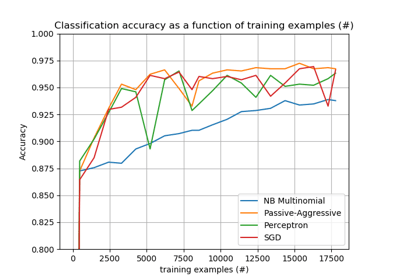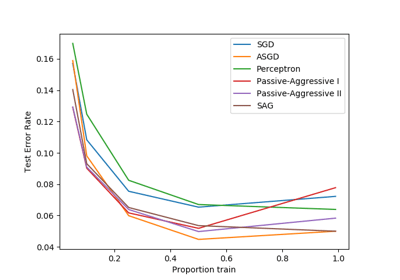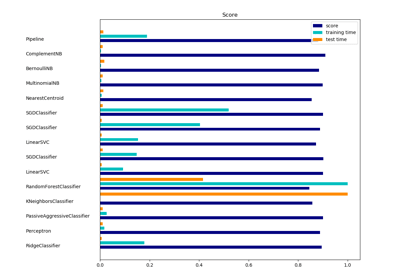/scikit-learn

# sklearn.linear_model.PassiveAggressiveClassifier

`class sklearn.linear_model.PassiveAggressiveClassifier(C=1.0, fit_intercept=True, max_iter=None, tol=None, early_stopping=False, validation_fraction=0.1, n_iter_no_change=5, shuffle=True, verbose=0, loss=’hinge’, n_jobs=None, random_state=None, warm_start=False, class_weight=None, average=False, n_iter=None)` [source]

Passive Aggressive Classifier

Read more in the User Guide.

Parameters: `C : float` Maximum step size (regularization). Defaults to 1.0. `fit_intercept : bool, default=False` Whether the intercept should be estimated or not. If False, the data is assumed to be already centered. `max_iter : int, optional` The maximum number of passes over the training data (aka epochs). It only impacts the behavior in the `fit` method, and not the `partial_fit`. Defaults to 5. Defaults to 1000 from 0.21, or if tol is not None. New in version 0.19. `tol : float or None, optional` The stopping criterion. If it is not None, the iterations will stop when (loss > previous_loss - tol). Defaults to None. Defaults to 1e-3 from 0.21. New in version 0.19. `early_stopping : bool, default=False` Whether to use early stopping to terminate training when validation. score is not improving. If set to True, it will automatically set aside a fraction of training data as validation and terminate training when validation score is not improving by at least tol for n_iter_no_change consecutive epochs. New in version 0.20. `validation_fraction : float, default=0.1` The proportion of training data to set aside as validation set for early stopping. Must be between 0 and 1. Only used if early_stopping is True. New in version 0.20. `n_iter_no_change : int, default=5` Number of iterations with no improvement to wait before early stopping. New in version 0.20. `shuffle : bool, default=True` Whether or not the training data should be shuffled after each epoch. `verbose : integer, optional` The verbosity level `loss : string, optional` The loss function to be used: hinge: equivalent to PA-I in the reference paper. squared_hinge: equivalent to PA-II in the reference paper. `n_jobs : int or None, optional (default=None)` The number of CPUs to use to do the OVA (One Versus All, for multi-class problems) computation. `None` means 1 unless in a `joblib.parallel_backend` context. `-1` means using all processors. See Glossary for more details. `random_state : int, RandomState instance or None, optional, default=None` The seed of the pseudo random number generator to use when shuffling the data. If int, random_state is the seed used by the random number generator; If RandomState instance, random_state is the random number generator; If None, the random number generator is the RandomState instance used by `np.random`. `warm_start : bool, optional` When set to True, reuse the solution of the previous call to fit as initialization, otherwise, just erase the previous solution. See the Glossary. Repeatedly calling fit or partial_fit when warm_start is True can result in a different solution than when calling fit a single time because of the way the data is shuffled. `class_weight : dict, {class_label: weight} or “balanced” or None, optional` Preset for the class_weight fit parameter. Weights associated with classes. If not given, all classes are supposed to have weight one. The “balanced” mode uses the values of y to automatically adjust weights inversely proportional to class frequencies in the input data as `n_samples / (n_classes * np.bincount(y))` New in version 0.17: parameter class_weight to automatically weight samples. `average : bool or int, optional` When set to True, computes the averaged SGD weights and stores the result in the `coef_` attribute. If set to an int greater than 1, averaging will begin once the total number of samples seen reaches average. So average=10 will begin averaging after seeing 10 samples. New in version 0.19: parameter average to use weights averaging in SGD `n_iter : int, optional` The number of passes over the training data (aka epochs). Defaults to None. Deprecated, will be removed in 0.21. Changed in version 0.19: Deprecated `coef_ : array, shape = [1, n_features] if n_classes == 2 else [n_classes, n_features]` Weights assigned to the features. `intercept_ : array, shape =  if n_classes == 2 else [n_classes]` Constants in decision function. `n_iter_ : int` The actual number of iterations to reach the stopping criterion. For multiclass fits, it is the maximum over every binary fit.

#### References

Online Passive-Aggressive Algorithms <http://jmlr.csail.mit.edu/papers/volume7/crammer06a/crammer06a.pdf> K. Crammer, O. Dekel, J. Keshat, S. Shalev-Shwartz, Y. Singer - JMLR (2006)

#### Examples

```>>> from sklearn.linear_model import PassiveAggressiveClassifier
>>> from sklearn.datasets import make_classification
>>>
>>> X, y = make_classification(n_features=4, random_state=0)
>>> clf = PassiveAggressiveClassifier(max_iter=1000, random_state=0)
>>> clf.fit(X, y)
PassiveAggressiveClassifier(C=1.0, average=False, class_weight=None,
early_stopping=False, fit_intercept=True, loss='hinge',
max_iter=1000, n_iter=None, n_iter_no_change=5, n_jobs=None,
random_state=0, shuffle=True, tol=None,
validation_fraction=0.1, verbose=0, warm_start=False)
>>> print(clf.coef_)
[[0.29509834 0.33711843 0.56127352 0.60105546]]
>>> print(clf.intercept_)
[2.54153383]
>>> print(clf.predict([[0, 0, 0, 0]]))

```

#### Methods

 `decision_function`(X) Predict confidence scores for samples. `densify`() Convert coefficient matrix to dense array format. `fit`(X, y[, coef_init, intercept_init]) Fit linear model with Passive Aggressive algorithm. `get_params`([deep]) Get parameters for this estimator. `partial_fit`(X, y[, classes]) Fit linear model with Passive Aggressive algorithm. `predict`(X) Predict class labels for samples in X. `score`(X, y[, sample_weight]) Returns the mean accuracy on the given test data and labels. `set_params`(*args, **kwargs) `sparsify`() Convert coefficient matrix to sparse format.
`__init__(C=1.0, fit_intercept=True, max_iter=None, tol=None, early_stopping=False, validation_fraction=0.1, n_iter_no_change=5, shuffle=True, verbose=0, loss=’hinge’, n_jobs=None, random_state=None, warm_start=False, class_weight=None, average=False, n_iter=None)` [source]
`decision_function(X)` [source]

Predict confidence scores for samples.

The confidence score for a sample is the signed distance of that sample to the hyperplane.

Parameters: `X : array_like or sparse matrix, shape (n_samples, n_features)` Samples. array, shape=(n_samples,) if n_classes == 2 else (n_samples, n_classes) Confidence scores per (sample, class) combination. In the binary case, confidence score for self.classes_ where >0 means this class would be predicted.
`densify()` [source]

Convert coefficient matrix to dense array format.

Converts the `coef_` member (back) to a numpy.ndarray. This is the default format of `coef_` and is required for fitting, so calling this method is only required on models that have previously been sparsified; otherwise, it is a no-op.

Returns: `self : estimator`
`fit(X, y, coef_init=None, intercept_init=None)` [source]

Fit linear model with Passive Aggressive algorithm.

Parameters: `X : {array-like, sparse matrix}, shape = [n_samples, n_features]` Training data `y : numpy array of shape [n_samples]` Target values `coef_init : array, shape = [n_classes,n_features]` The initial coefficients to warm-start the optimization. `intercept_init : array, shape = [n_classes]` The initial intercept to warm-start the optimization. `self : returns an instance of self.`
`get_params(deep=True)` [source]

Get parameters for this estimator.

Parameters: `deep : boolean, optional` If True, will return the parameters for this estimator and contained subobjects that are estimators. `params : mapping of string to any` Parameter names mapped to their values.
`loss_function`

DEPRECATED: Attribute loss_function was deprecated in version 0.19 and will be removed in 0.21. Use `loss_function_` instead

`partial_fit(X, y, classes=None)` [source]

Fit linear model with Passive Aggressive algorithm.

Parameters: `X : {array-like, sparse matrix}, shape = [n_samples, n_features]` Subset of the training data `y : numpy array of shape [n_samples]` Subset of the target values `classes : array, shape = [n_classes]` Classes across all calls to partial_fit. Can be obtained by via `np.unique(y_all)`, where y_all is the target vector of the entire dataset. This argument is required for the first call to partial_fit and can be omitted in the subsequent calls. Note that y doesn’t need to contain all labels in `classes`. `self : returns an instance of self.`
`predict(X)` [source]

Predict class labels for samples in X.

Parameters: `X : array_like or sparse matrix, shape (n_samples, n_features)` Samples. `C : array, shape [n_samples]` Predicted class label per sample.
`score(X, y, sample_weight=None)` [source]

Returns the mean accuracy on the given test data and labels.

In multi-label classification, this is the subset accuracy which is a harsh metric since you require for each sample that each label set be correctly predicted.

Parameters: `X : array-like, shape = (n_samples, n_features)` Test samples. `y : array-like, shape = (n_samples) or (n_samples, n_outputs)` True labels for X. `sample_weight : array-like, shape = [n_samples], optional` Sample weights. `score : float` Mean accuracy of self.predict(X) wrt. y.
`sparsify()` [source]

Convert coefficient matrix to sparse format.

Converts the `coef_` member to a scipy.sparse matrix, which for L1-regularized models can be much more memory- and storage-efficient than the usual numpy.ndarray representation.

The `intercept_` member is not converted.

Returns: `self : estimator`

#### Notes

For non-sparse models, i.e. when there are not many zeros in `coef_`, this may actually increase memory usage, so use this method with care. A rule of thumb is that the number of zero elements, which can be computed with `(coef_ == 0).sum()`, must be more than 50% for this to provide significant benefits.

After calling this method, further fitting with the partial_fit method (if any) will not work until you call densify.

## Examples using `sklearn.linear_model.PassiveAggressiveClassifier`Out-of-core classification of text documentsComparing various online solversClassification of text documents using sparse features